# HSSlive: Plus One & Plus Two Notes & Solutions for Kerala State Board

## AP Board Class 7 Maths Chapter 11 Exponents Ex 1 Textbook Solutions PDF: Download Andhra Pradesh Board STD 7th Maths Chapter 11 Exponents Ex 1 Book AnswersAP Board Class 7 Maths Chapter 11 Exponents Ex 1 Textbook Solutions PDF: Download Andhra Pradesh Board STD 7th Maths Chapter 11 Exponents Ex 1 Book Answers

## Andhra Pradesh State Board Class 7th Maths Chapter 11 Exponents Ex 1 Books Solutions

 Board AP Board Materials Textbook Solutions/Guide Format DOC/PDF Class 7th Subject Maths Chapters Maths Chapter 11 Exponents Ex 1 Provider Hsslive

2. Click on the Andhra Pradesh Board Class 7th Maths Chapter 11 Exponents Ex 1 Answers.
3. Look for your Andhra Pradesh Board STD 7th Maths Chapter 11 Exponents Ex 1 Textbooks PDF.

## AP Board Class 7th Maths Chapter 11 Exponents Ex 1 Textbooks Solutions with Answer PDF Download

Find below the list of all AP Board Class 7th Maths Chapter 11 Exponents Ex 1 Textbook Solutions for PDF’s for you to download and prepare for the upcoming exams:

Question 1.
Write the base and the exponent in each case. Also, write the term in the expanded form.
(i) 34
(ii) (7x)2
(iii) (5ab)3
(iv) (4y)5
Solution: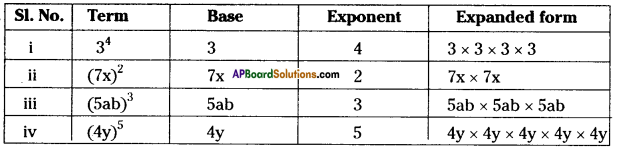Question 2.
Write the exponential form of each expression.
(i) 7 × 7 × 7 × 7 × 7
(ii) 3 × 3 × 3 × 5 × 5 × 5 × 5
(iii) 2 × 2 × 2 × 3 × 3 × 3 × 3 × 5 × 5 × 5
Solution:
(i) 7 × 7 × 7 × 7 × 7 = 75
(ii) 3 × 3 × 3 × 5 × 5 × 5 × 5 = 33 × 54
(iii) 2 × 2 × 2 × 3 × 3 × 3 × 3 × 5 × 5 × 5 = 23 × 34 × 53

Question 3.
Express the following as the product of exponents through prime factorization.
(i) 288
(ii) 1250
(iii) 2250
(iv) 3600
(v) 2400
Solution:
(i) 288
1) 288 = 2 × 144
= 2 × 2 × 72
= 2 × 2 × 2 × 36
= 2 × 2 × 2 × 2 × 18
= 2 × 2 × 2 × 2 × 2 × 9
= (2 × 2 × 2 × 2× 2) × (3 × 3)
= 25 × 32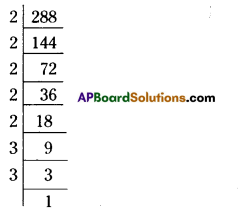(ii) 1250 = 2 × 625
= 2 × 5 × 125
= 2 × 5 × 5 × 25
= 2 × 5 × 5 × 5 × 5
= 2 × 54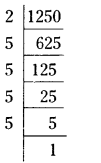(iii) 2250 = 2 × 1125
= 2 × 3 × 375
= 2 × 3 × 3 × 125
= 2 × 3 × 3 × 5 × 25
= 2 × 3 × 3 × 5 × 5 × 5
= 21 × 32 × 53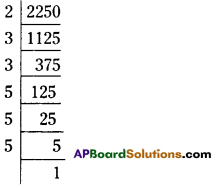(iv) 3600 = 2 × 1800
= 2 × 2 × 900
=2 × 2 × 2 × 450
=2 × 2 × 2 × 2 × 225
= ( 2 × 2 × 2 × 2 ) × 3 × 75
= (2 × 2 × 2 × 2) × (3 × 3) × 25
= (2 × 2 × 2 × 2) × (3 × 3) × (5 × 5)
= 24 × 32 × 52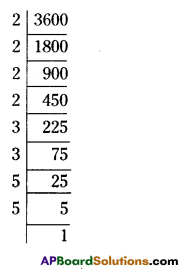(v) 2400
2400 = 2 × 1200
= 2 × 2 × 600
= 2 × 2 × 2 × 300
= 2 × 2 × 2 × 2 × 150
= 2 × 2 × 2 × 2 × 2 × 75
= (2 × 2 × 2 × 2 × 2) × 3 × 25
= (2 × 2 × 2 × 2 × 2) × 3 × (5 × 5)
= 25 × 31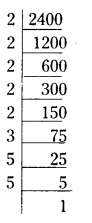Question 4.
Identify the greater number in each of the following pairs.
(i) 23 or 32
(ii) 53 or 35
(iii) 28 or 82
Solution:
(i) 23 or 32 = 23 = 2 × 2 × 2 = 8 and 32 = 3 × 3 = 9
∴ 23 < 32 or 32 > 23

(ii) 53 or 35 = 53 = 5 × 5 × 5 = 125 and 35 = 3 × 3 × 3 × 3 × 3 = 243
∴ 35 > 53

(iii) 28 or 82 = 28 = 2 × 2 × 2 × 2 × 2 × 2 × 2 × 2 = 256
82 = 8 × 8 = 64
∴ 28 > 82

Question 5.
If a = 3, b = 2 find the value of
(i) ab + ba
(ii) aa + bb
(iii) (a + b)ab
(iv)(a – b)a
Solution:
(i) ab + ba = 32 + 23 = 3 × 3 + 2 × 2 × 2 = 9 + 8 =17
(ii) aa + bb = 33 + 22 = 3 × 3 × 3 + 2 × 2 = 27 + 4 = 31
(iii) (a + b)b = (3 + 2)2 = 52 = 5 × 5 = 25
(iv)(a – b)a = (3 – 2)2= 12 = 1 × 1 = 1 .

## Andhra Pradesh Board Class 7th Maths Chapter 11 Exponents Ex 1 Textbooks for Exam Preparations

Andhra Pradesh Board Class 7th Maths Chapter 11 Exponents Ex 1 Textbook Solutions can be of great help in your Andhra Pradesh Board Class 7th Maths Chapter 11 Exponents Ex 1 exam preparation. The AP Board STD 7th Maths Chapter 11 Exponents Ex 1 Textbooks study material, used with the English medium textbooks, can help you complete the entire Class 7th Maths Chapter 11 Exponents Ex 1 Books State Board syllabus with maximum efficiency.

## FAQs Regarding Andhra Pradesh Board Class 7th Maths Chapter 11 Exponents Ex 1 Textbook Solutions

#### Can we get a Andhra Pradesh State Board Book PDF for all Classes?

Yes you can get Andhra Pradesh Board Text Book PDF for all classes using the links provided in the above article.

## Important Terms

Andhra Pradesh Board Class 7th Maths Chapter 11 Exponents Ex 1, AP Board Class 7th Maths Chapter 11 Exponents Ex 1 Textbooks, Andhra Pradesh State Board Class 7th Maths Chapter 11 Exponents Ex 1, Andhra Pradesh State Board Class 7th Maths Chapter 11 Exponents Ex 1 Textbook solutions, AP Board Class 7th Maths Chapter 11 Exponents Ex 1 Textbooks Solutions, Andhra Pradesh Board STD 7th Maths Chapter 11 Exponents Ex 1, AP Board STD 7th Maths Chapter 11 Exponents Ex 1 Textbooks, Andhra Pradesh State Board STD 7th Maths Chapter 11 Exponents Ex 1, Andhra Pradesh State Board STD 7th Maths Chapter 11 Exponents Ex 1 Textbook solutions, AP Board STD 7th Maths Chapter 11 Exponents Ex 1 Textbooks Solutions,
Share: